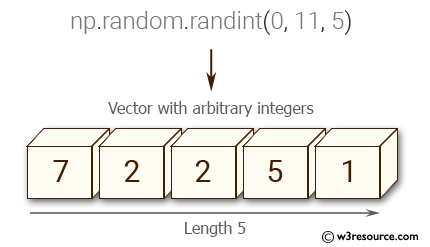﻿ NumPy: Create a vector of length 5 filled with arbitrary integers from 0 to 10 - w3resource# NumPy: Create a vector of length 5 filled with arbitrary integers from 0 to 10

## NumPy: Basic Exercise-23 with Solution

Write a NumPy program to create a vector of length 5 filled with arbitrary integers from 0 to 10.

Sample Solution :

Python Code :

``````import numpy as np
x = np.random.randint(0, 11, 5)
print("Vector of length 5 filled with arbitrary integers from 0 to 10:")
print(x)
``````

Sample Output:

```Vector of length 5 filled with arbitrary integers from 0 to 10:
[ 0 10  2  0  6]
```

Pictorial Presentation:Python Code Editor:

Have another way to solve this solution? Contribute your code (and comments) through Disqus.

What is the difficulty level of this exercise?

Test your Python skills with w3resource's quiz

﻿

## Python: Tips of the Day

Checks if the given number falls within the given range.

Example:

```def tips_range(n, start, end = 0):
return start <= n <= end if end >= start else end <= n <= start
print(tips_range(2, 4, 6))
print(tips_range(4, 8))
print(tips_range(1, 3, 5))
print(tips_range(1, 3))
```

Output:

```False
True
False
True
```